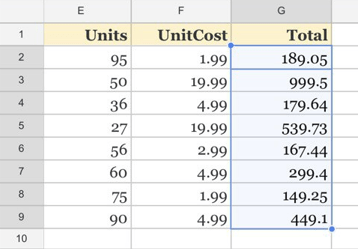# How to☝️ Copy a Formula Down an Entire Column in Google Sheets

To copy a formula down an entire column in Google Sheets, select the entire column with the initial result you calculated and press the Ctrl + D key combination on your keyboard. If you need to copy a formula down a column quickly, this is the way to do it!

## Method 1: Using AutoFill

AutoFill is a feature in Google Sheets that allows you to quickly copy formulas or data down an entire column or across multiple rows. This can be extremely helpful when you’re working with large amounts of data and need to perform calculations on all of it. Let’s take a look at how it’s done.

Difficulty: Beginner

Time Estimate: 5 seconds

1. Select the cell where you want the first result of the formula.
2. Type the formula. In the example below, we use E2*F2.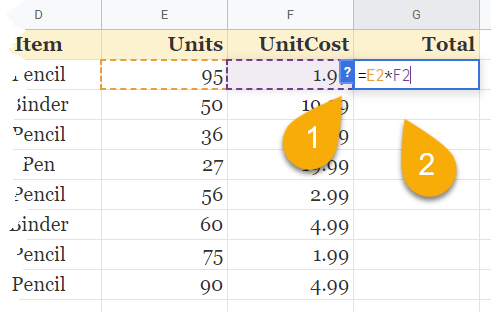1. Hit Enter to display the result.
2. When the AutoFill suggestion appears, tap on the check mark to fill in the rest of the values.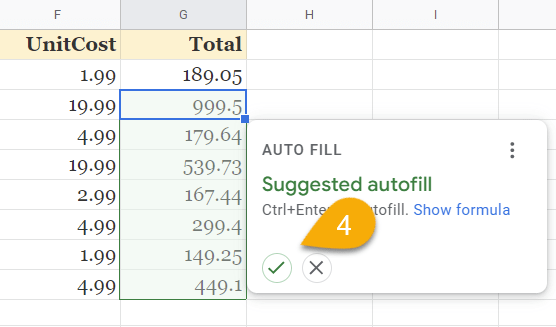Piece of cake! Google Sheets makes it super easy.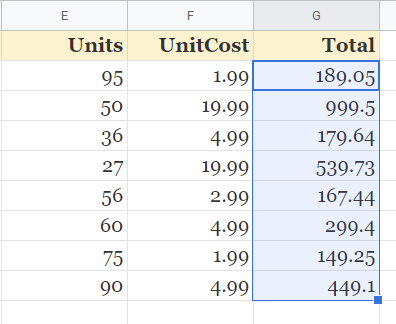## Method 2: Dragging the Fill Handle

This is another very easy method you can use to copy down a formula through a list of data. With this method, you can manually select which cells will apply the formula.

Difficulty: Beginner

Time Estimate: 5 seconds

1. Click on the cell where you want the initial result of the formula.
2. Enter the formula you need.1. Press the Enter key on your keyboard.
2. Click on the small square icon in the lower right corner of the cell and drag it downward through the rest of the list.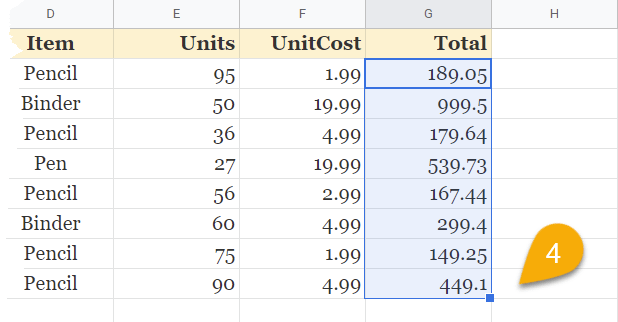Voila! The formula has been copied into the selected cells.

## Method 3: Using the ARRAY Formula

The ARRAY Formula is a powerful formula that can be used in various situations. In this section, we will show you how to use the ARRAY formula to copy a formula down an entire column in Google Sheets easily. Let’s check it out!

Difficulty: Beginner

Time Estimate: 10 seconds

1. Click on the cell where you want the initial value from the formula.
2. Enter the formula =ArrayFormula(E2:E9*F2:F9), where E2:E9 and F2:F9 are ranges of the values you need to calculate.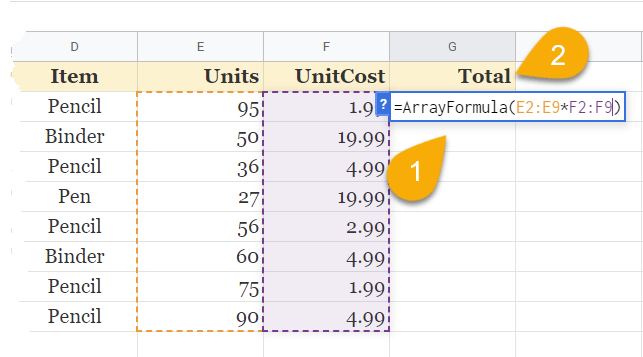1. Tap the Enter key.And here is the result!

## Copying a Formula Down FAQs

For more information on how to copy the formula down a list of data, please take a look at the FAQs below.

### How do I repeat a formula down a column in Google Sheets without dragging the cell?

In this method, you will be able to copy the formula down a column by using the keyboard instead of dragging. To do it, select a blank cell and type the formula you need.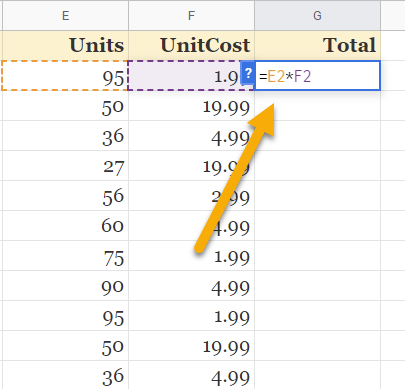Hit Enter to get the result. Then highlight the cells you want to copy the formula into and press the Ctrl + D (Windows) / Cmd + D (Mac OS) key combination.

### How do I copy a formula down thousands of rows?

To copy a formula down thousands of rows in one simple action, start by clicking on the cell where the result needs to be displayed and enter the formula in that cell.Next, press the Enter key on the keyboard to get the result. Then double-click the icon in the lower right corner of the cell.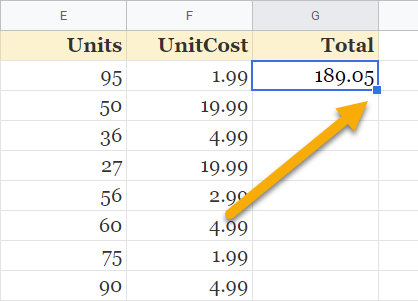This action will copy the formula through the entire list, no matter how long it is.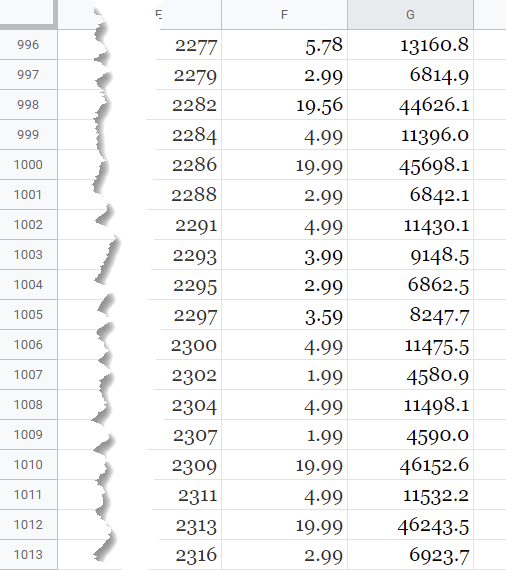### How do I copy a formula down without changing cell references?

To copy the formula down without changing cell references, select the cell where you want the result. Next, type your formula using dollar signs to lock the cell reference so it doesn’t change. You can also press the F4 key on your keyboard after entering each cell reference to make the dollar signs appear automatically. In our example, we use the formula =\$E\$2*\$F\$2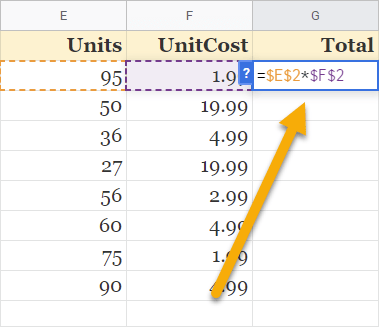Press the Enter key to get the result and drag the cell with the result down.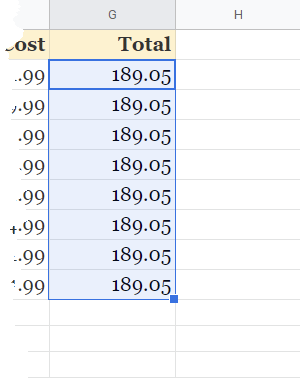### How do I copy a formula down in Google Sheets on mobile?

To copy the formula down in Google Sheets on mobile, first click on the cell where you want to add the formula. Go to the Formula bar at the bottom of the screen and enter your desired formula there. Once you’ve entered your formula, tap the checkmark icon to save it.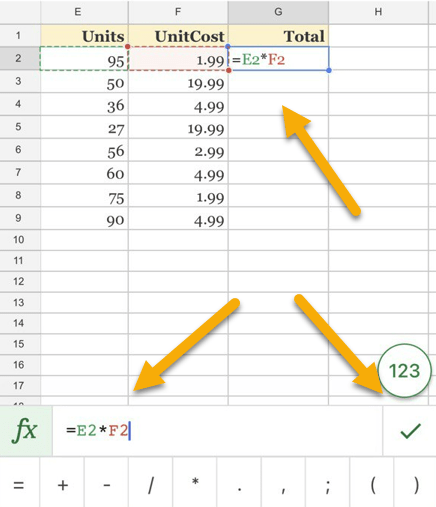Next, select the column where you need to copy the formula. Click on the selected range and pick the AutoFill option.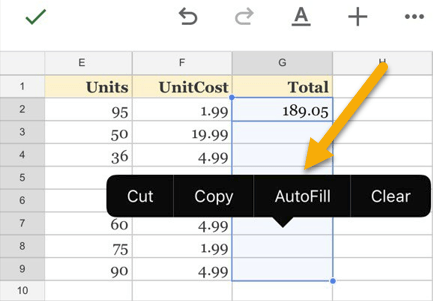Your formula will now be copied down the column!